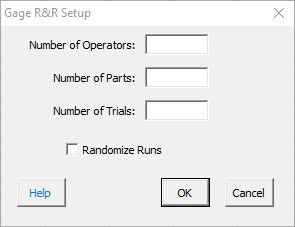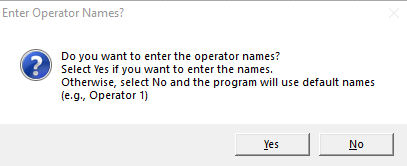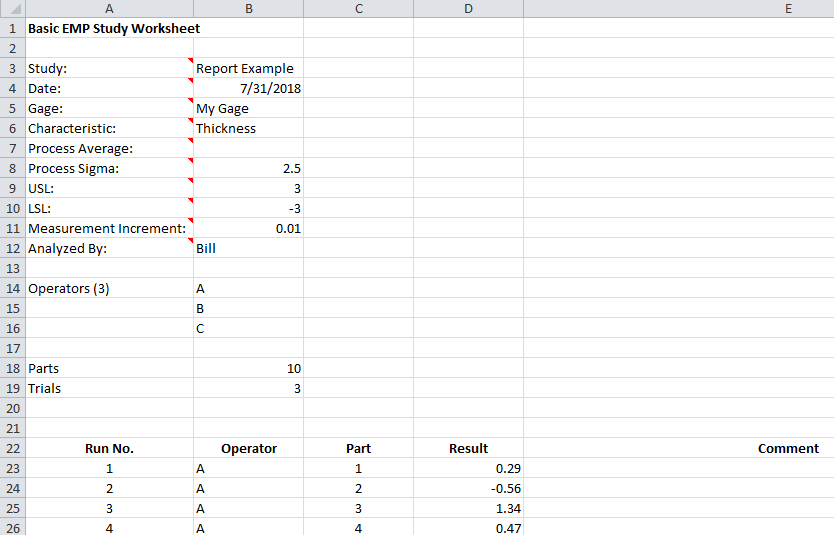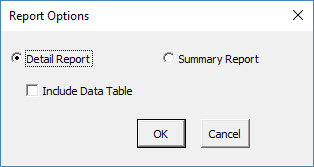# Basic EMP Study HelpIn a Basic EMP study, multiple operators repeatably  measure multiple parts.  The setup is a classical crossed Gage R&R analysis.

This help page describes how to perform the EMP consistency study using data that be downloaded at this link.

1. To start the analysis, select “MSA” from the “Analysis” panel on the SPC for Excel ribbon.  Then select “EMP” and “Basic EMP Study”.

2. The input form below is shown.  Enter the number of operators, parts and trials.  You also have the option to randomize the runs in the study. Select OK.3. The option to enter the operators’ names is given.  If you select No, the software lists each operator as Operator 1, Operator 2, etc.  If you select yes, then an input form is shown for each operator so you can enter the name.4. A Basic EMP Study worksheet is added to the workbook. This is where you enter the information and results.5. Enter the information into the top part of the worksheet.  There is a comment in each cell in rows 3 to 12 in column A that explain the entry.  The only required entry is the measurement increment.  Each entry is explained below.

• Study: optional,name of study
• Date: optional, date Gage R&R performed
• Gage: optional, name of gage
• Characteristic: optional, characteristic being measured
• Process Average: optional
• Used to calculate Cpk for one-sided specs for the class of monitor
• Process Sigma: optional, value used for the total variance instead of the calculated total variance of the Gage R&R results
• USL: optional, used to calculate the Cp or Cpk values for the class of monitor; used to determine manufacturing specs if measurement increment is entered
• LSL: optional, used to calculate the Cp or Cpk values for the class of monitor; used to determine manufacturing specs if measurement increment is entered
• Measurement Increment: required, used to determine if measurement increment is adequate based on probable error; used to determine manufacturing specs if specs are entered
• Analyzed By: optional, name of person doing the analysis

6. Enter the run number, the sample result for each combination of operator and part and any comments (optional).

7. Part of a completed short EMP worksheet is shown below.8. The results are now ready to be analyzed using the EMP consistency method. To analyze the results, select “MSA” from the “Analysis” panel on the SPC for Excel ribbon.

9. The following options are displayed.  You have an option for detailed report or a summary report, both displayed on single worksheet. The detailed report contains all the charts, calculations and explanation of results.  The summary report contains the charts.  You also have the option to include a data table in the report.  Select OK to generate the report in the workbook.10. A pdf file containing the detailed report with a description of the output is given at this link.  The output includes:

• X-R chart
• Control chart calculations
• X chart analysis
• R chart analysis
• Main effects chart
• Mean range chart
• ANOM calculations
• Main effects chart analysis
• Mean range chart analysis
• Repeatability (test-retest error)
• Probable error and measurement increment
• Variance components
• Intraclass correlation coefficient
• Type of class monitor
• Watershed specifications and precision to tolerance ratio
• Data table